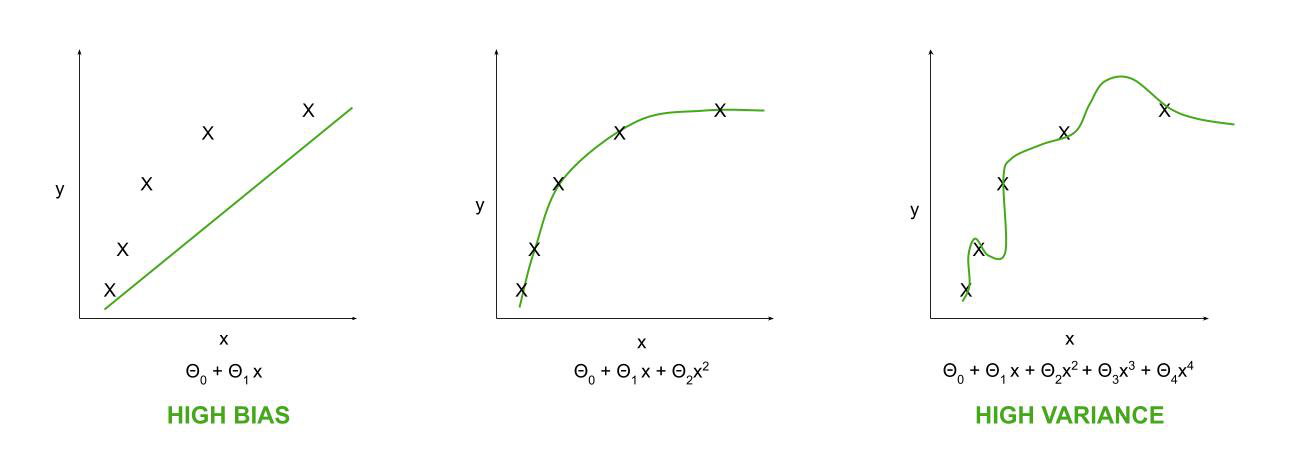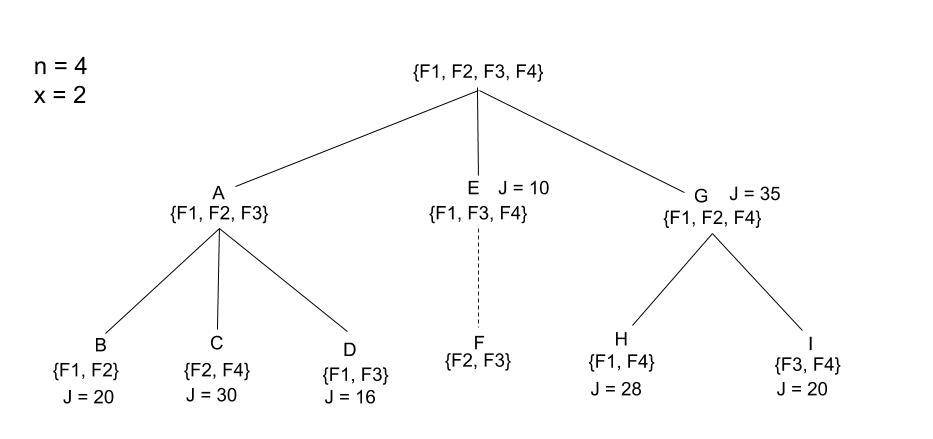# Feature Selection using Branch and Bound Algorithm

• Last Updated : 26 Nov, 2020

Feature selection is a very important factor in Machine Learning. To get the algorithms to work properly and give near about perfect predictions, i.e to enhance the performance of a predictive model, feature selection is required. Large set of features or redundant ones should be removed. Up to a certain number of features, the accuracy of a classifier increases but there is a threshold after which it starts to decrease. Using too many or too few features can lead to the problem of high variance and high bias. So, searching for the best subset of features is very important.

If dimension is large, i.e. a large set of features is used then a large data set is also recommended.

To remove the problem of high variance:

```Use a smaller set of features.
```

To remove the problem of high bias:

```Use additional features.
```Learning Curves with different feature sets for a single data set

It is to be found out manually what the maximum number of features will be to form the best subset with the highest criterion function or cost function, J.

Branch and Bound Algorithm:

This algorithm is typically used in the supervised learning algorithm. It follows a tree structure to select the best subset of features.

The root node consists of all features, say n. The intermediate children nodes consist of features one less than their parent node and the sequence is followed until the leaf nodes are reached. Once the feature subset size has been fixed, say x, then the tree can be drawn with leaf nodes having x features. Once a leaf node has been reached, the criterion value for it is evaluated and set as a bound value, b. On further evaluation of other branches, if its criterion value exceeds b then b is updated to it and that branch is expanded to its leaf nodes. If its criterion value does not exceed b then that branch is skipped. Ultimately, the leaf node with the highest criterion value is selected.

Let’s understand the algorithm with an example.Branch and Bound tree with n = 4 and x = 2

Here, the parent node has all the 4 features. In the next level, it is branched into 3 children nodes who have one feature less than the root node. Each of these nodes is again broken into their children nodes and ultimately to the leaf node level where the leaf nodes are the final feature subsets of size 2, one of which will be selected.

It is important to note the naming of the nodes here because that is how the tree has been created. Node A gives rise to nodes B, C and D. Once we get the leaf node B, we evaluate its criterion value first. Here, J = 20. So, bound value, b is set to 20. Now, node C is generated and it’s J = 30 which is higher than b so b gets updated to 30 and the best feature subset gets updated to node C. Next, node D is generated and its J = 16 which is lesser than that of C, so b remains unchanged.

Now, the second child of the root node, E is created and its J = 10 which is again lesser than C, so it is not further expanded as this subset combination cannot give us a better criterion value for our model. So, node F is not created and its value is also not required to be checked. This is how we reduce the cost of modeling and also the time spent on it.

Now, the third and final child of the root node, G is generated and it’s J = 35 which is higher than C’s so it is expanded. Node H and I have J value 28 and 20 respectively, both of which are not higher than C so {F2, F4} is the best feature subset for this problem.

My Personal Notes arrow_drop_up